### The Unreasonable Effectiveness of Quasirandom Sequences

I present a new low discrepancy quasirandom sequence that offers many substantial improvements over other popular sequences such as the Sobol and Halton sequences.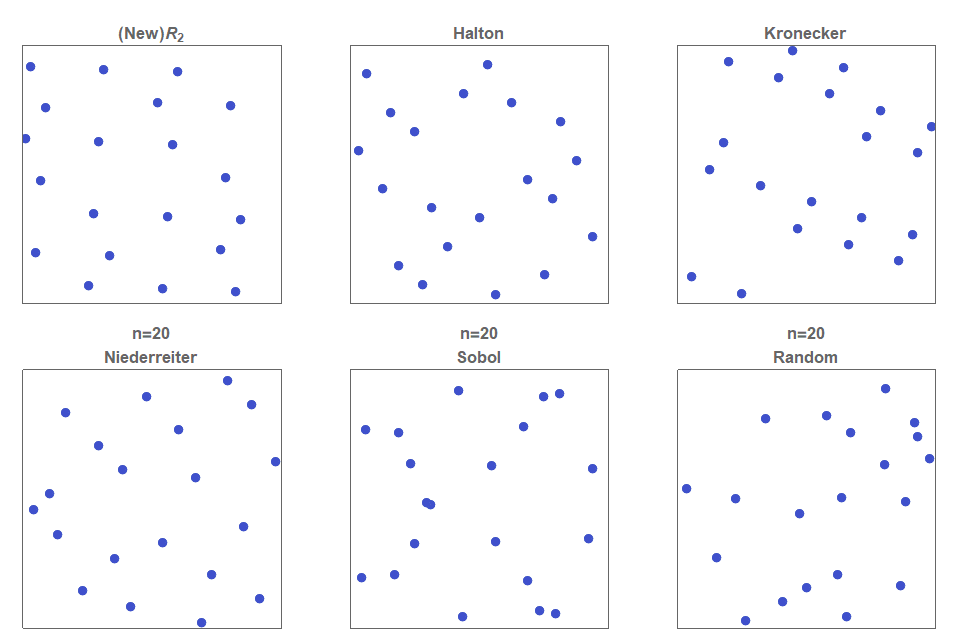Figure 1a. Comparison of the various low discrepancy quasirandom sequences. Note that the newly proposed $R$-sequence produces more evenly spaced points than any of the other methods. Furthermore, all other current methods require careful selection of basis parameters, and if not chosen carefully can lead to degeneracy (eg top right).

### A new algorithm for multi-armed bandits

I propose a new algorithm for multi-armed bandits, that is fully deterministic, as fast and simple to implement as UCB1, and yet, for a very broad range of practical circumstances empirically outperforms many of the existing industry standard Bernoulli multi-armed bandit policies, including Thompson Sampling and UCB-tuned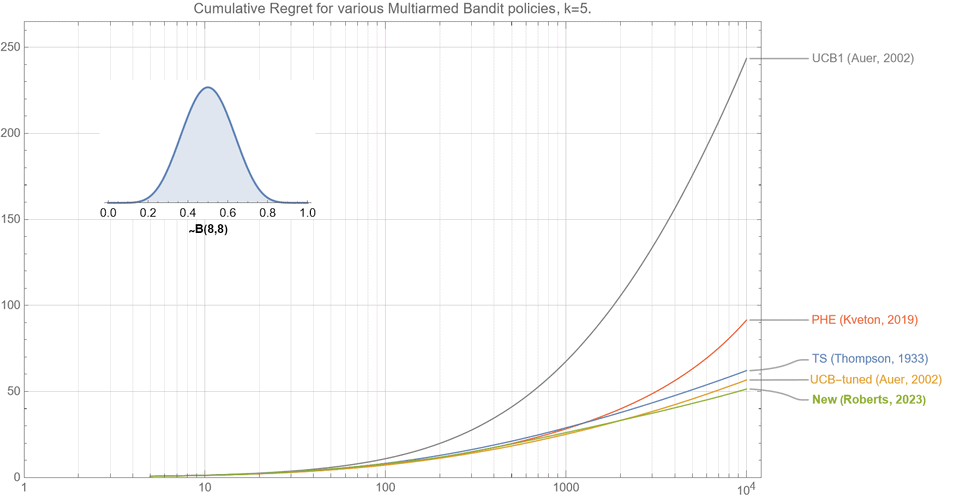Figure 1. This comparison of various bandit policies shows that if the underlying probabilities for the $k=5$ arms are drawn from a beta distribution $B(8,8)$, then the cumulative regret curve (smaller is better) for our new algorithm (as described in section 2.5) outperforms UCB1, UCB-tuned, Perturbed History Exploration (PHE), and Thompson Sampling for almost all $t<10^4$.

### How to evenly distribute points on a sphere more effectively than the canonical Fibonacci Lattice

Mapping the Fibonacci lattice (aka Golden Spiral, aka Fibonacci Sphere) onto the surface of a sphere is an extremely fast and effective approximate method to evenly distribute points on a sphere. I show how small modifications to the canonical implementation can result in notable improvements for nearest-neighbor measures.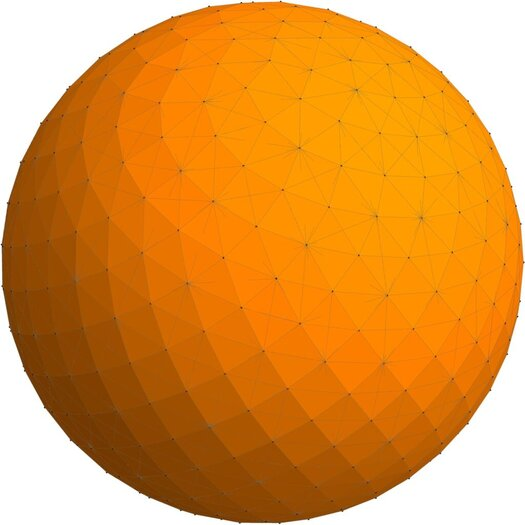Figure 1. A simple modification to the canonical Fibonacci lattice can result in an improvement of up to 8.3 Continue reading “How to evenly distribute points on a sphere more effectively than the canonical Fibonacci Lattice”

### How to generate uniformly random points on n-spheres and in n-balls

For many Monte Carlo methods, such as those in graphical computing, it is critical to uniformly sample from $d$-dimensional spheres and balls. This post describes over twenty different methods to uniformly random sample from the (surface of) a $d$-dimensional sphere or the (interior of) a $d$-dimensional ball.

Continue reading “How to generate uniformly random points on n-spheres and in n-balls”

### Going beyond the Golden Ratio.

I show that for the same reason that the golden ratio, $\phi=1.6180334..$, can be considered the most irrational number, that $1+\sqrt{2}$ can be considered the 2nd most irrational number, and indeed why $(9+\sqrt{221})/10$ can be considered the 3rd most irrational number.

Continue reading “Going beyond the Golden Ratio.”

### Maximal Poisson disk sampling: an improved version of Bridson’s algorithm

Bridson’s Algorithm (2007) is a very popular method to produce maximal ‘blue noise’ sample point distributions such that no two points are closer than a specified distance apart. In this brief post we show how a minor modification to this algorithm can make it up to 20x faster and allows it to produce much higher density blue noise sample point distributions.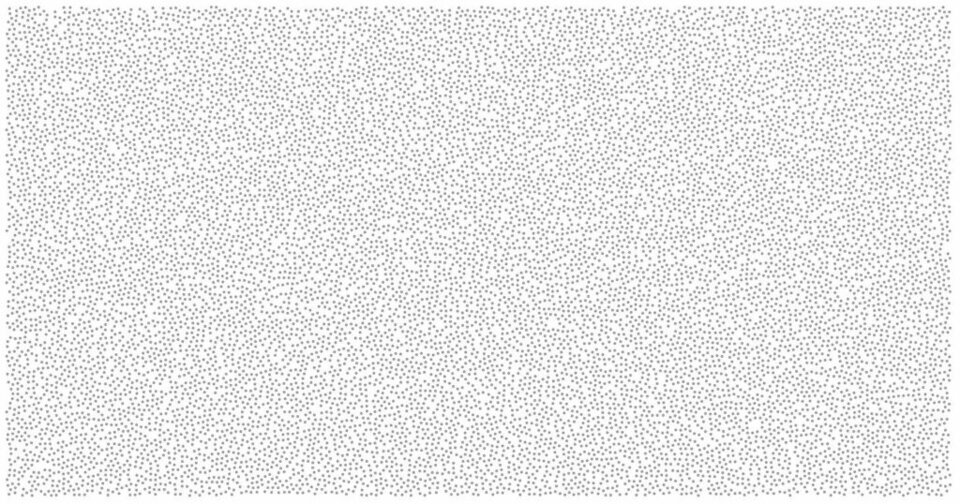Figure 1. Poisson disc sampling based on a modified version of Bridson’s algorithm. This modified algorithm runs in linear time and is up to 20x faster than the original algorithm

Continue reading “Maximal Poisson disk sampling: an improved version of Bridson’s algorithm”

### A new method to construct isotropic blue-noise sample point sets with uniform projections

I describe a how a small but critical modification to correlated multi-jittered sampling can significantly improve its blue noise spectral characteristics whilst maintaining its uniform projections. This is an exact and direct grid-based construction method that guarantees a minimum neighbor point separation of at least $0.707/n$ and has an average point separation of $0.965/n$.Figure 1. Examples of point sets with $n^2$ points for $n=8,12,16,24,32,40$. The minimum nearest neighbor distance is guaranteed to exceed $0.707/n$ and the average distance is $0.965/n$. Their blue noise sample point distributions are isotropic and their 1-D projections are exactly uniformly distributed. Futhermore each set can be directly and exactly constructed through just two permutations of the $n$-dimensional vector $\{1,2,3,…,n\}$. This therefore, presents a new way of directly constructing tileable isotropic blue noise without the need for iterative methods such as best candidate or simulated annealing

Continue reading “A new method to construct isotropic blue-noise sample point sets with uniform projections”

### A simple method to construct isotropic quasirandom blue noise point sequences

I describe a simple method for constructing a sequence of points, that is based on a low discrepancy quasirandom sequence but exhibits enhanced isotropic blue noise properties. This results in fast convergence rates with minimal aliasing artifacts.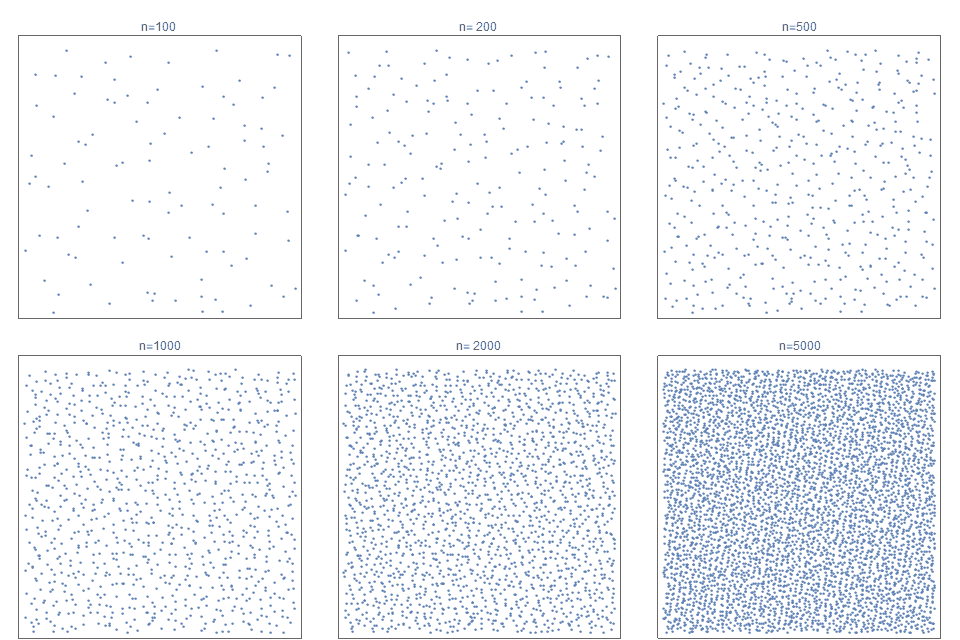Figure 1. The first 100, 200, 500, 1000, 2000 and 5000 sample points of the proposed point sequence (eqn 11) that is progressive, non-stochastic, exhibits near isotropic blue noise characteristics with fast QMC convergence rates with reduced artifacts. It is based on a new simple low discrepancy quasirandom sequence, $R_2$.

Continue reading “A simple method to construct isotropic quasirandom blue noise point sequences”

### Evenly Distributing Points in a Triangle.

Most  two dimensional quasirandom methods focus on sampling over a unit square. However, sampling evenly over the triangle is also very important in computer graphics.  Therefore,  I describe a simple and direct construction method for a point sequence to evenly cover an arbitrary shaped triangle.Figure 1. A new direct method for constructing an open (infinite) low discrepancy quasirandom sequence over an triangle of arbitrary shape and size. Shown are the point distributions for twelve random triangles for the first 150 points.

Continue reading “Evenly Distributing Points in a Triangle.”

### An alternate canonical grid layout with uniform projections

When points are placed in a canonical grid layout, they are well-separated and their projections are uniform. I present a simple canonical grid layout which  offers better closest-neighbor characterisics than the two most common contemporary canonical layouts.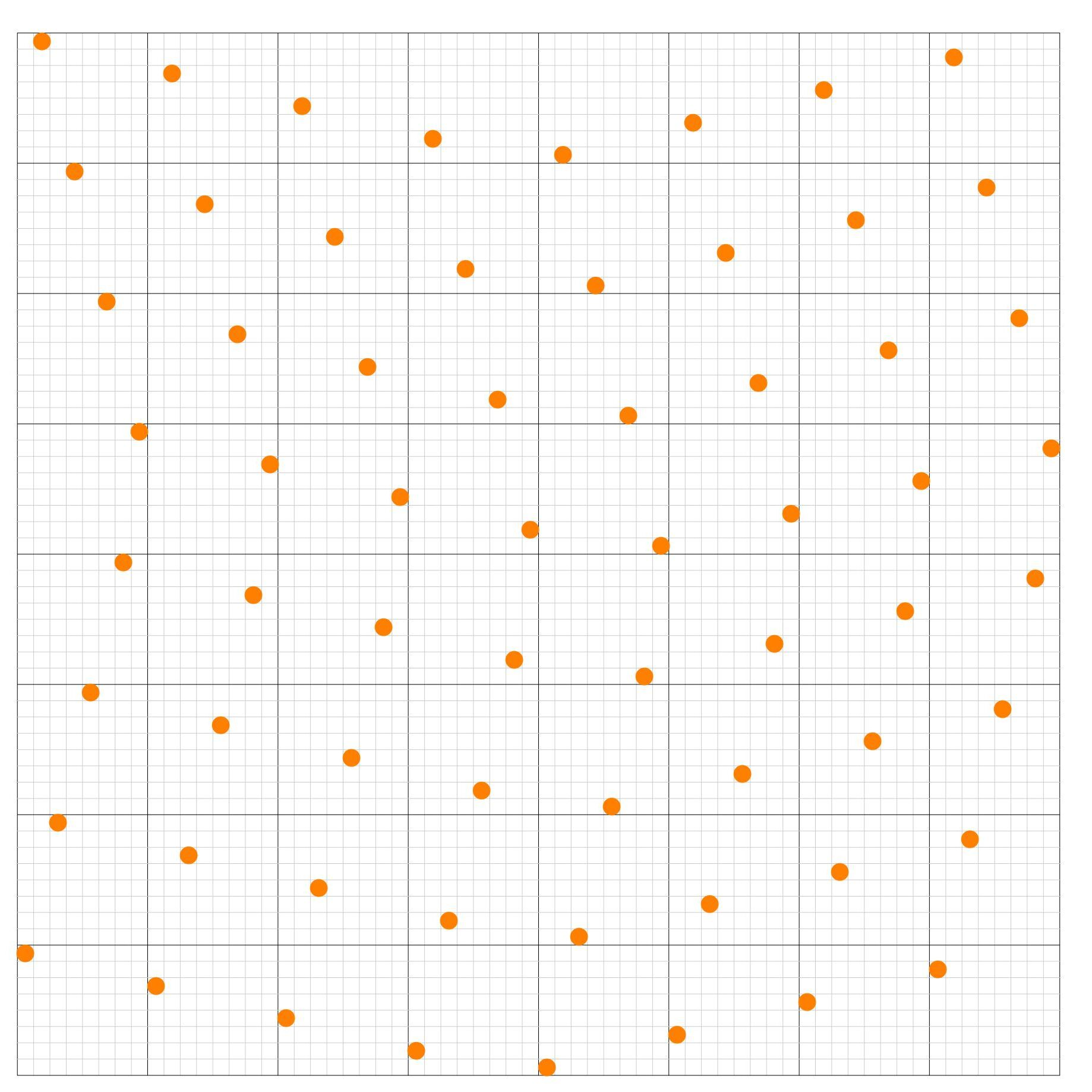Figure 1. A canonical grid layout whose projections are uniform and its closest neighbor distance characteristics are optimal.

Continue reading “An alternate canonical grid layout with uniform projections”

### Trigonometry in Pictures

This post shows how the core trigonometric definitions, relations and addition theorems can be simply and intuitively visualized.

### A Formula for the Perimeter of an Ellipse

Unlike for circles, there isn’t a simple exact closed formula for the perimeter of an ellipse. We compare several well-known approximations, and conclude that a formula discovered by Ramanujan is our favourite, due to its simplicity and extreme accuracy.Continue reading “A Formula for the Perimeter of an Ellipse”

### Lissajous Curves

This post illustrates and explains the beautiful Lissajous Curves – trajectories of points whose coordinates follow sinusoidal movements.  A simple but timeless classic curve that has numerous applications as well as artistic elegance.### A simple formula for Sequences and Series

If you are given the initial terms of a sequence, then here is an insanely simple method to derive a general formula for the first N terms, as well as the sum of the first N terms of the sequence.Continue reading “A simple formula for Sequences and Series”

### A probabilistic approach to fractional factorial design

I describe a probabilistic alternative to fractional factorial design based on the Sobol’ low discrepancy quasirandom sequence. This method is robust to aliasing  (confounders), is often simpler to implement than traditional fractional factorial sample designs, and produces more accurate results than simple random sampling.Continue reading “A probabilistic approach to fractional factorial design”

### The Theil-Sen and Siegel non-parametric estimators for linear regression

The Theil-Sen (Kendall) and Siegel estimators are non-parametric distribution-free methods used to fit a line to data, in ways that are very robust to large levels of noise and outliers. We briefly illustrate how the lesser-known Siegel estimator is typically better than the more commonly used Theil-Sen estimator.

Continue reading “The Theil-Sen and Siegel non-parametric estimators for linear regression”

### Multiple Pendulums

This fun post illustrates the phenomenon of multiple uncoupled pendulums whose periods are all rational multiples of each other.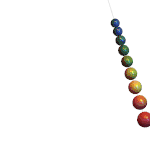Figure 1. Multiple uncoupled pendulums. All periods are rationally related. This entire motion has a period of precisely 60 seconds.

### The Fisher-Yates Algorithm

Creating unbiased random permutations of lists is often crucial to sampling. The Fisher-Yates shuffle is the definitive method to shuffle a sequence of items. Popularised by Knuth, it is unbiased, has optimal linear time efficiency; uses constant space; and is incremental.